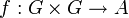# Cyclicity-preserving 2-cocycle for trivial group action

## Definition

Suppose$G$ is a group and$A$ is an abelian group. A function$f:G \times G \to A$ is termed a cyclicity-preserving 2-cocycle for trivial group action if it satisfies the following conditions:

Condition name Expression for condition
2-cocycle for a group action (particularly, 2-cocycle for trivial group action)$\! f(g_2,g_3) + f(g_1,g_2g_3) = f(g_1g_2,g_3) + f(g_1,g_2)$ for all$g_1,g_2,g_3 \in G$
cyclicity-preserving$\! f(g,h) = 0$ whenever$\langle g,h \rangle$ is cyclic

The group of cyclicity-preserving 2-cocycles is denoted$Z^2_{CP}(G,A)$.

## Facts

### Existence of a source group

For any group$G$, there exists an abelian group$K$ such that for any abelian group$A$, the group of cyclicity-preserving 2-cocycles$\! f:G \times G \to A$ can be identified with the group$\operatorname{Hom}(K,A)$.

## Examples

### Extreme examples

• If$G$ is a cyclic group or a locally cyclic group, the group of cyclicity-preserving 2-cocycles is a trivial group.
• If$A$ is a trivial group, the group of cyclicity-preserving 2-cocycles is a trivial group.

## Relation with other properties

### Weaker properties

Property Meaning Proof of implication Proof of strictness (reverse implication failure) Intermediate notions
IIP 2-cocycle for trivial group action 2-cocycle$f$ such that$\! f(g,1) = f(1,g) = f(g,g^{-1}) = 0$ for all$g \in G$ |FULL LIST, MORE INFO
normalized 2-cocycle for trivial group action 2-cocycle$f$ such that$\! f(g,1) = f(1,g) = 0$ for all$g \in G$ |FULL LIST, MORE INFO
2-cocycle for trivial group action IIP 2-cocycle for trivial group action|FULL LIST, MORE INFO